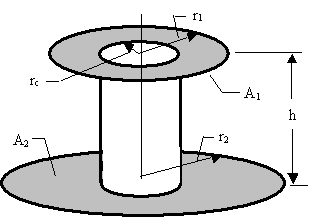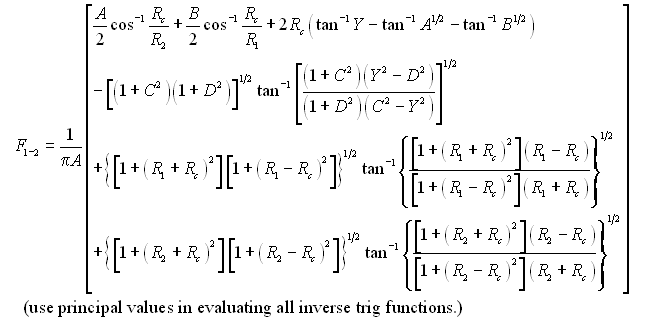# SECTION C Factors From Finite Areas to Finite Areas

## C-53: Annulus to coaxial annulus of different outer radius; both annuli have inner radius of blocking coaxial cylinder.

Reference: Brockmann; Bornside and BrownDefinitions:

 R1 = r1/h; R2 = r2/h; Rc = rc/h; A = R12-Rc2; B = R22-Rc2; C=R2+R1; D=R2-R1; Y=A1/2+B1/2

Governing Equation:To download a copy of this equation in MS Word 97 Format, click HERE.